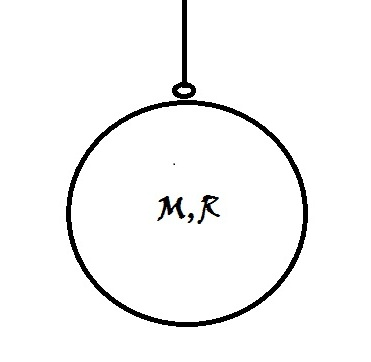# Infinite PendulumFind the Time period of oscillation (in sec) of an infinite pendulum, which is hovering just above the surface of the Earth.

Note: An Infinite Pendulum is a obviously infinitely long. The Hinge of the Pendulum is at infinite distance from the surface of the Earth.
Also, note that the all the mass of the pendulum is concentrated at the bottom of the pendulum, where the bob is situated (in other words, the mass is not uniformly distributed...Its more like a stone tied at the end of a massless string)

Details and Assumptions:
$\bullet$ Assume that the oscillations are very small.
$\bullet$ $\displaystyle g_{surface} = 9.8m/s^2$
$\bullet$ $\displaystyle R_{Earth} = 6400Km$

×

Problem Loading...

Note Loading...

Set Loading...